1. 单变量函数的泰勒公式

[泰勒局部公式]  如果函数f(x)满足条件:(i)在点a的某邻域内有定义,(ii)在此邻域内有一直到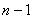阶的导数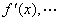,,(iii)在点a处有n阶导数,那末f(x)在点a的邻域内可表成以下各种形式:

f (a+h)= f (a)+=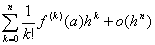(h0)

f (x)= f (a)+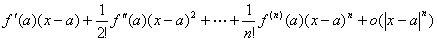=(xa)

[马克劳林公式]

f (x)= f (0)+=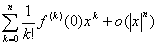(x0)

[泰勒公式]  如果函数f (x)满足条件:(i)在闭区间[a,b]上有定义,(ii)在此闭区间上有一直到n阶的连续导数,(iii)a<x<b时有有限导数,那末f(x)在闭区间[a,b]上可表成以下各种形式:

f(a+h)=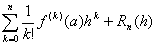(a<a+h<b)

Rn(h)=(0<θ<1)   (柯西型余项)

f(x)=()

Rn(x)=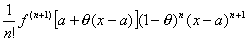(0<θ<1)    (柯西型余项)

[马克劳林公式]

f(x)=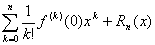(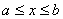)

Rn(x)=(0<θ<1)     (柯西型余项)

[泰勒级数]  在带余项的泰勒公式,如果把展开式进行到(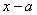)的任意高的乘幂,则有

f(x)=f(a)+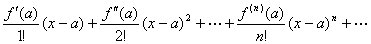f(a),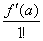,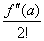,…,,…

[马克劳林级数]  在带余项的马克劳林公式中,如果展开式进行到x的任意高的乘幂,则有

f(x)=f(0)+f (0),,,…,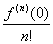,…